# Python | Pandas Series.combine()

• Last Updated : 17 Feb, 2019

Pandas series is a One-dimensional ndarray with axis labels. The labels need not be unique but must be a hashable type. The object supports both integer- and label-based indexing and provides a host of methods for performing operations involving the index.

Pandas` Series.combine()` function combine the Series with a Series or scalar according to func. It combine the Series and other using func to perform element-wise selection for combined Series. fill_value is assumed when value is missing at some index from one of the two objects being combined.

Syntax: Series.combine(other, func, fill_value=None)

Parameter :
other : Series or scalar
func : Function that takes two scalars as inputs and returns an element.
fill_value : The value to assume when an index is missing from one Series or the other.

Returns : Series

Example #1: Use `Series.combine()` function to find the maximum value for each index labels in the two series object.

 `# importing pandas as pd ` `import` `pandas as pd ` ` `  `# Creating the first Series ` `sr1 ``=` `pd.Series([``80``, ``25``, ``3``, ``25``, ``24``, ``6``]) ` ` `  `# Creating the second Series ` `sr2 ``=` `pd.Series([``34``, ``5``, ``13``, ``32``, ``4``, ``15``]) ` ` `  `# Create the Index ` `index_ ``=` `[``'Coca Cola'``, ``'Sprite'``, ``'Coke'``, ``'Fanta'``, ``'Dew'``, ``'ThumbsUp'``] ` ` `  `# set the first index ` `sr1.index ``=` `index_ ` ` `  `# set the second index ` `sr2.index ``=` `index_ ` ` `  `# Print the first series ` `print``(sr1) ` ` `  `# Print the second series ` `print``(sr2) `

Output :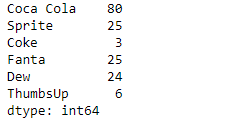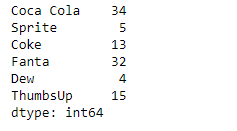Now we will use `Series.combine()` function to find the maximum value for each index labels in the two given series object.

 `# find the maximum element-wise ` `# among sr1 and sr2 ` `result ``=` `sr1.combine(other ``=` `sr2, func ``=` `max``) ` ` `  `# Print the result ` `print``(result) `

Output :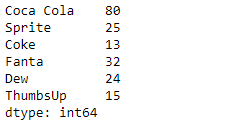As we can see in the output, the `Series.combine()` function has successfully returned the maximum value for each index labels among the two series objects.

Example #2 : Use `Series.combine()` function to find the minimum value for each index labels in the two series object.

 `# importing pandas as pd ` `import` `pandas as pd ` ` `  `# Creating the first Series ` `sr1 ``=` `pd.Series([``51``, ``10``, ``24``, ``18``, ``None``, ``84``, ``12``, ``10``, ``5``, ``24``, ``2``]) ` ` `  `# Creating the second Series ` `sr2 ``=` `pd.Series([``11``, ``21``, ``8``, ``18``, ``65``, ``18``, ``32``, ``10``, ``5``, ``32``, ``None``]) ` ` `  `# Create the Index ` `index_ ``=` `pd.date_range(``'2010-10-09'``, periods ``=` `11``, freq ``=``'M'``) ` ` `  `# set the first index ` `sr1.index ``=` `index_ ` ` `  `# set the second index ` `sr2.index ``=` `index_ ` ` `  `# Print the first series ` `print``(sr1) ` ` `  `# Print the second series ` `print``(sr2) `

Output :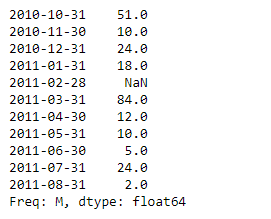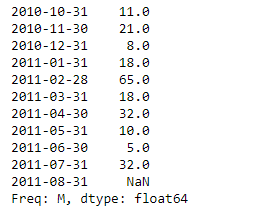Now we will use `Series.combine()` function to find the minimum value for each index labels in the two given series object.

 `# find the minimum element-wise ` `# among sr1 and sr2 ` `result ``=` `sr1.combine(other ``=` `sr2, func ``=` `min``) ` ` `  `# Print the result ` `print``(result) `

Output :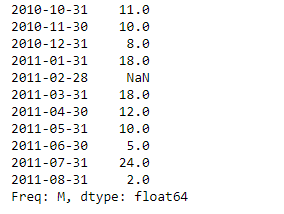As we can see in the output, the `Series.combine()` function has successfully returned the minimum value for each index labels among the two series objects.

My Personal Notes arrow_drop_up
Recommended Articles
Page :